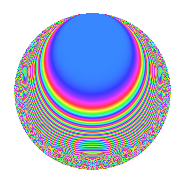# Properties

 Label 105.3.lLevel 105 Weight 3 Character orbit l Rep. character $$\chi_{105}(22,\cdot)$$ Character field $$\Q(\zeta_{4})$$ Dimension 24 Newform subspaces 1 Sturm bound 48 Trace bound 0

# Related objects

## Defining parameters

 Level: $$N$$ $$=$$ $$105 = 3 \cdot 5 \cdot 7$$ Weight: $$k$$ $$=$$ $$3$$ Character orbit: $$[\chi]$$ $$=$$ 105.l (of order $$4$$ and degree $$2$$) Character conductor: $$\operatorname{cond}(\chi)$$ $$=$$ $$5$$ Character field: $$\Q(i)$$ Newform subspaces: $$1$$ Sturm bound: $$48$$ Trace bound: $$0$$

## Dimensions

The following table gives the dimensions of various subspaces of $$M_{3}(105, [\chi])$$.

Total New Old
Modular forms 72 24 48
Cusp forms 56 24 32
Eisenstein series 16 0 16

## Trace form

 $$24q + 8q^{2} + 16q^{5} + 24q^{6} - 48q^{8} + O(q^{10})$$ $$24q + 8q^{2} + 16q^{5} + 24q^{6} - 48q^{8} - 40q^{10} - 48q^{12} + 64q^{13} - 184q^{16} + 24q^{17} + 24q^{18} + 72q^{20} + 8q^{22} + 8q^{23} - 136q^{25} - 80q^{26} + 96q^{30} + 96q^{31} + 56q^{32} - 72q^{33} + 168q^{36} + 8q^{37} + 56q^{38} + 232q^{40} + 320q^{41} - 112q^{43} - 72q^{45} + 320q^{46} + 64q^{47} + 192q^{48} - 256q^{50} - 192q^{51} + 96q^{52} - 72q^{53} - 80q^{55} - 336q^{56} + 48q^{57} - 512q^{58} - 192q^{60} - 496q^{61} - 776q^{62} + 312q^{65} - 192q^{66} - 192q^{67} + 568q^{68} + 112q^{70} - 144q^{71} + 144q^{72} + 224q^{73} + 144q^{75} + 416q^{76} + 112q^{77} - 216q^{78} - 528q^{80} - 216q^{81} + 352q^{82} - 32q^{83} + 24q^{85} + 240q^{86} + 384q^{87} + 216q^{88} - 24q^{90} + 1304q^{92} + 376q^{95} + 168q^{96} - 816q^{97} - 56q^{98} + O(q^{100})$$

## Decomposition of $$S_{3}^{\mathrm{new}}(105, [\chi])$$ into newform subspaces

Label Dim. $$A$$ Field CM Traces $q$-expansion
$$a_2$$ $$a_3$$ $$a_5$$ $$a_7$$
105.3.l.a $$24$$ $$2.861$$ None $$8$$ $$0$$ $$16$$ $$0$$

## Decomposition of $$S_{3}^{\mathrm{old}}(105, [\chi])$$ into lower level spaces

$$S_{3}^{\mathrm{old}}(105, [\chi]) \cong$$ $$S_{3}^{\mathrm{new}}(15, [\chi])$$$$^{\oplus 2}$$$$\oplus$$$$S_{3}^{\mathrm{new}}(35, [\chi])$$$$^{\oplus 2}$$

## Hecke characteristic polynomials

There are no characteristic polynomials of Hecke operators in the database